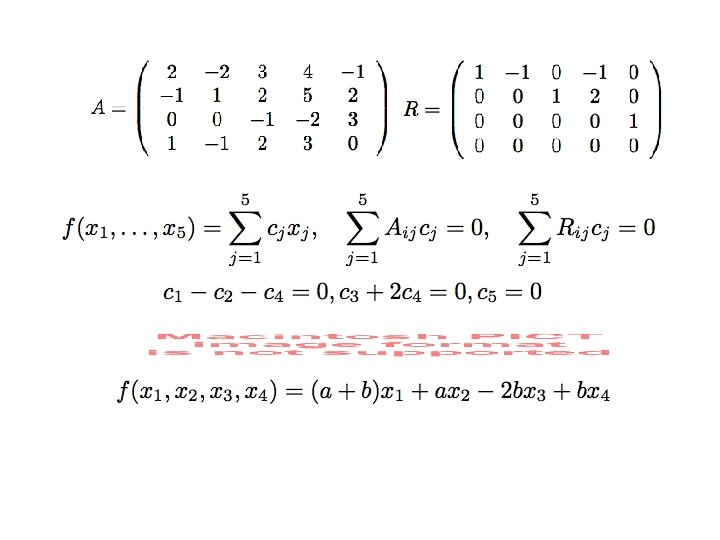# Representation by matrices Representation Basis change T VnWm

• Slides: 21Representation by matrices Representation. Basis change.• T: Vn->Wm.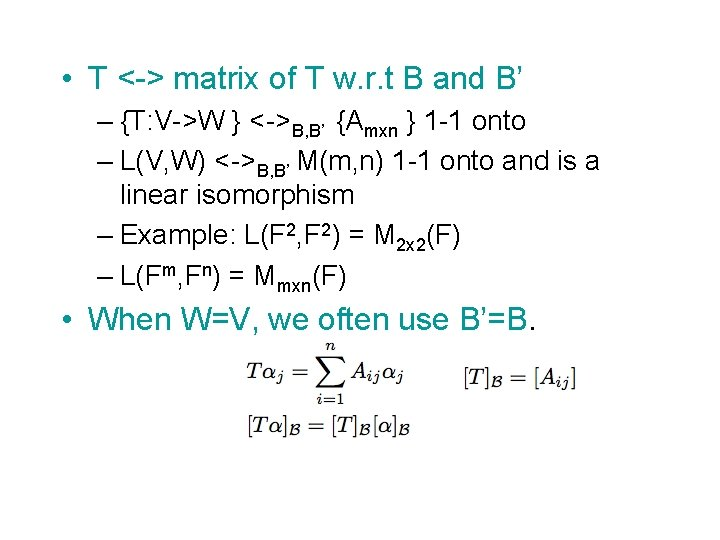• T <-> matrix of T w. r. t B and B’ – {T: V->W } <->B, B’ {Amxn } 1 -1 onto – L(V, W) <->B, B’ M(m, n) 1 -1 onto and is a linear isomorphism – Example: L(F 2, F 2) = M 2 x 2(F) – L(Fm, Fn) = Mmxn(F) • When W=V, we often use B’=B.• Example: V = Fnx 1, W=Fmx 1, – T: V->W defined by T(X)=AX. – B, B’ standard basis – Then [T]B, B’ = A.• Theorem: V, W, Z, T: V->W, U: W->Z basis B, B’, B”, A=[T]B, B’, B=[U]B’, B”. Then C = AB = [U T]B, B’’. – Matrix multiplication correspond to compositions. • Corollary: [UT]B=[U]B[T]B when V=W=Z, B=B’=B”. • Corollary. [T-1]B = ([T]B)-1 – Proof: UT=I=TU. U=T-1. [U]B[T]B=[I]B=[T]B[U]B.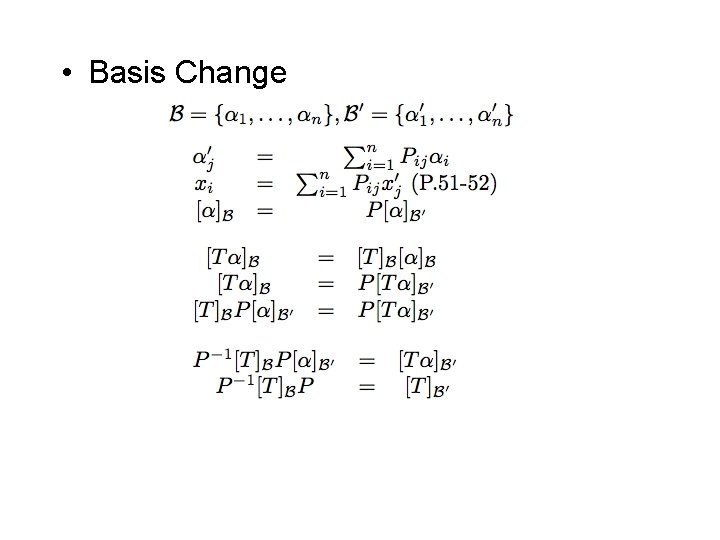• Basis Change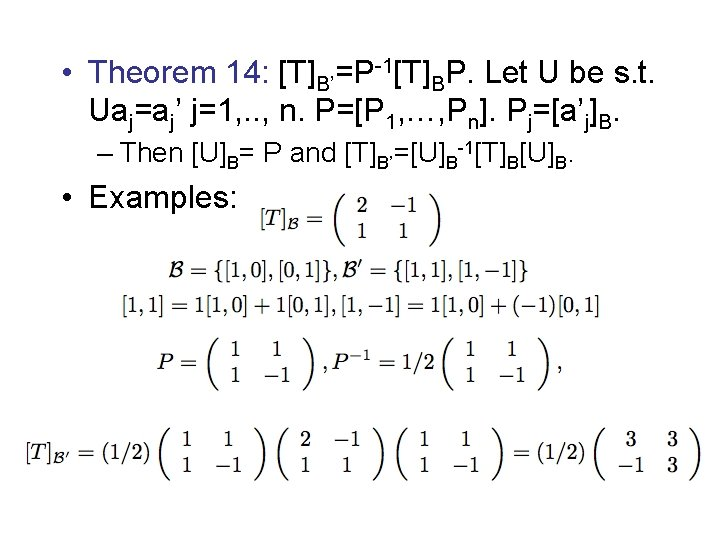• Theorem 14: [T]B’=P-1[T]BP. Let U be s. t. Uaj=aj’ j=1, . . , n. P=[P 1, …, Pn]. Pj=[a’j]B. – Then [U]B= P and [T]B’=[U]B-1[T]B[U]B. • Examples:• Example: – B is a basis – Define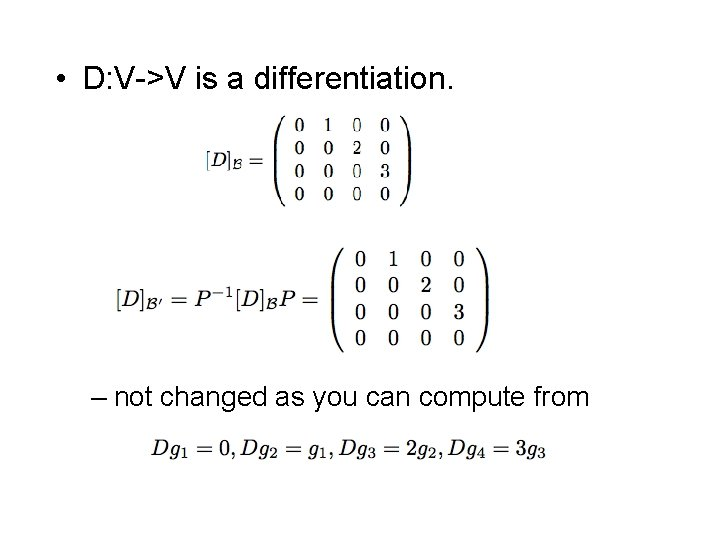• D: V->V is a differentiation. – not changed as you can compute from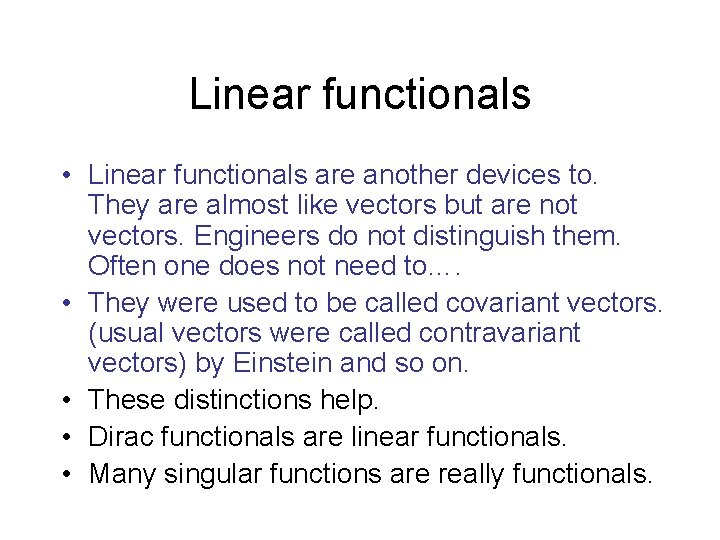Linear functionals • Linear functionals are another devices to. They are almost like vectors but are not vectors. Engineers do not distinguish them. Often one does not need to…. • They were used to be called covariant vectors. (usual vectors were called contravariant vectors) by Einstein and so on. • These distinctions help. • Dirac functionals are linear functionals. • Many singular functions are really functionals.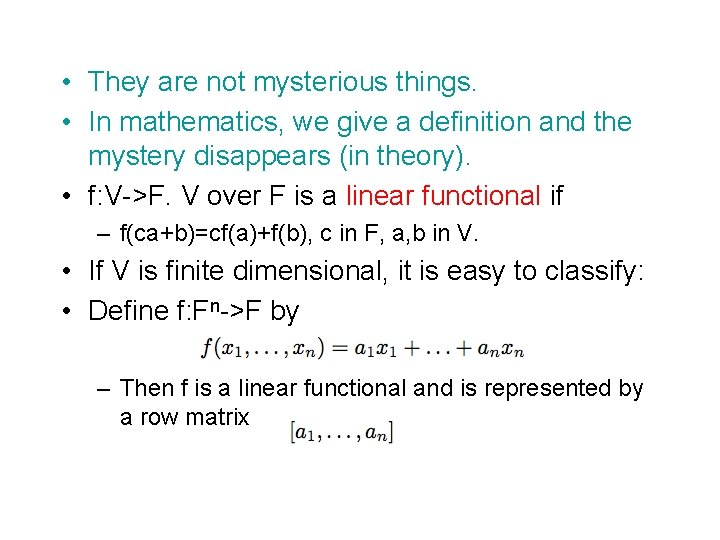• They are not mysterious things. • In mathematics, we give a definition and the mystery disappears (in theory). • f: V->F. V over F is a linear functional if – f(ca+b)=cf(a)+f(b), c in F, a, b in V. • If V is finite dimensional, it is easy to classify: • Define f: Fn->F by – Then f is a linear functional and is represented by a row matrix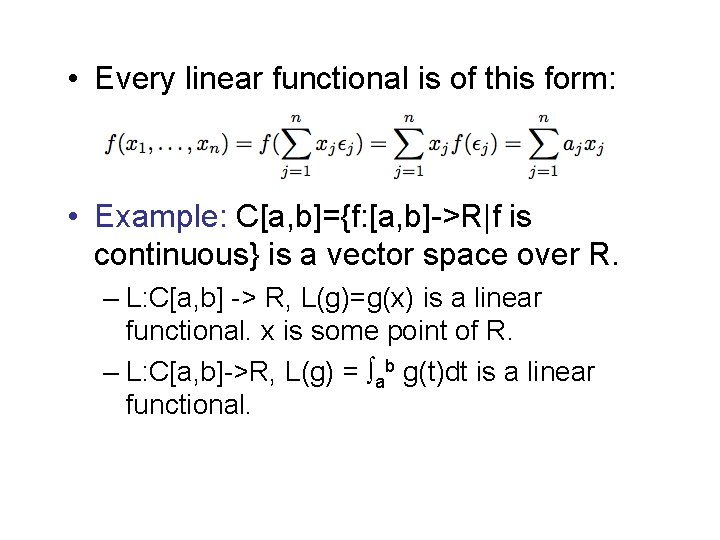• Every linear functional is of this form: • Example: C[a, b]={f: [a, b]->R|f is continuous} is a vector space over R. – L: C[a, b] -> R, L(g)=g(x) is a linear functional. x is some point of R. – L: C[a, b]->R, L(g) = ab g(t)dt is a linear functional.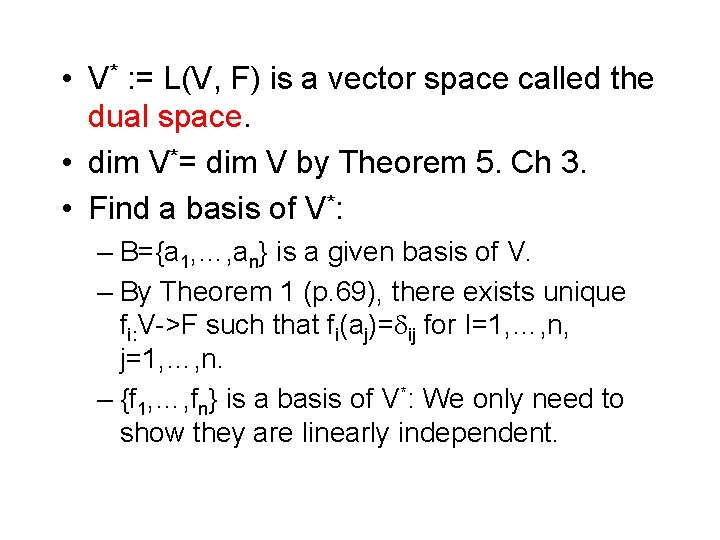• V* : = L(V, F) is a vector space called the dual space. • dim V*= dim V by Theorem 5. Ch 3. • Find a basis of V*: – B={a 1, …, an} is a given basis of V. – By Theorem 1 (p. 69), there exists unique fi: V->F such that fi(aj)= ij for I=1, …, n, j=1, …, n. – {f 1, …, fn} is a basis of V*: We only need to show they are linearly independent.• Proof: • B*={f 1, …, fn} is the dual basis of V*. • Theorem 15: – (1) B* is a basis. (2) – (3) – Proof: (1) done. (2) from (*)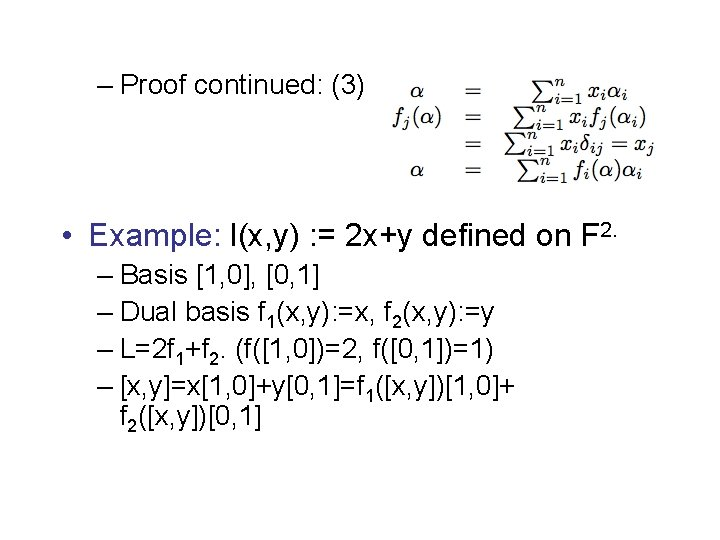– Proof continued: (3) • Example: l(x, y) : = 2 x+y defined on F 2. – Basis [1, 0], [0, 1] – Dual basis f 1(x, y): =x, f 2(x, y): =y – L=2 f 1+f 2. (f([1, 0])=2, f([0, 1])=1) – [x, y]=x[1, 0]+y[0, 1]=f 1([x, y])[1, 0]+ f 2([x, y])[0, 1]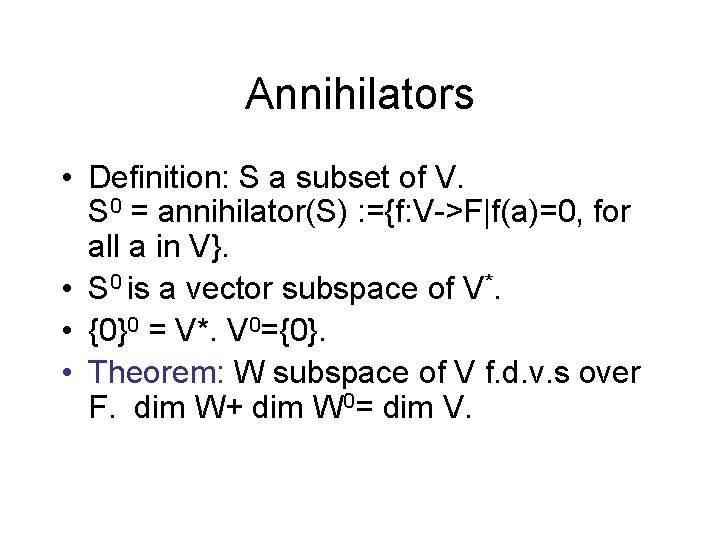Annihilators • Definition: S a subset of V. S 0 = annihilator(S) : ={f: V->F|f(a)=0, for all a in V}. • S 0 is a vector subspace of V*. • {0}0 = V*. V 0={0}. • Theorem: W subspace of V f. d. v. s over F. dim W+ dim W 0= dim V.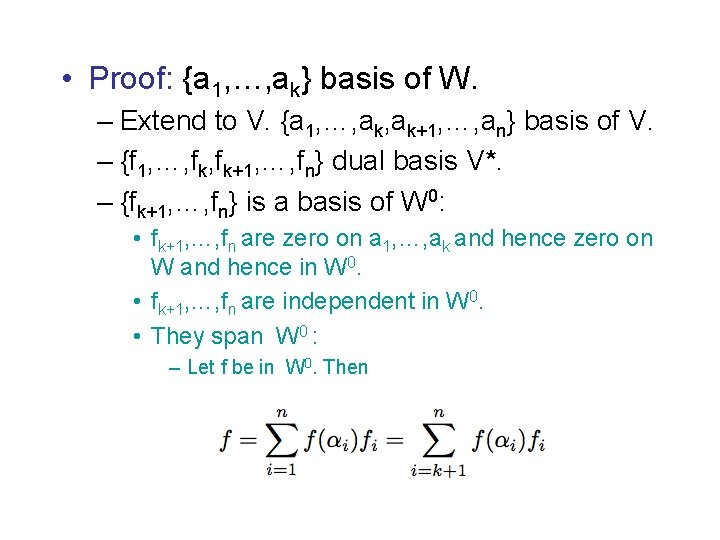• Proof: {a 1, …, ak} basis of W. – Extend to V. {a 1, …, ak+1, …, an} basis of V. – {f 1, …, fk+1, …, fn} dual basis V*. – {fk+1, …, fn} is a basis of W 0: • fk+1, …, fn are zero on a 1, …, ak and hence zero on W and hence in W 0. • fk+1, …, fn are independent in W 0. • They span W 0 : – Let f be in W 0. Then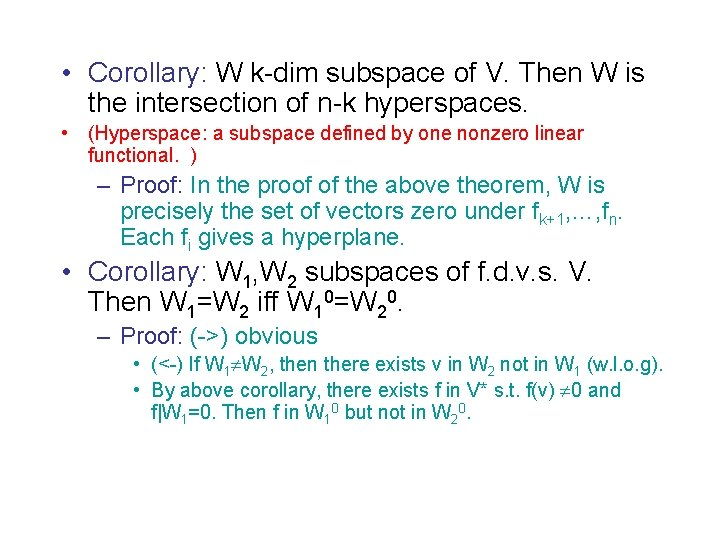• Corollary: W k-dim subspace of V. Then W is the intersection of n-k hyperspaces. • (Hyperspace: a subspace defined by one nonzero linear functional. ) – Proof: In the proof of the above theorem, W is precisely the set of vectors zero under fk+1, …, fn. Each fi gives a hyperplane. • Corollary: W 1, W 2 subspaces of f. d. v. s. V. Then W 1=W 2 iff W 10=W 20. – Proof: (->) obvious • (<-) If W 1 W 2, then there exists v in W 2 not in W 1 (w. l. o. g). • By above corollary, there exists f in V* s. t. f(v) 0 and f|W 1=0. Then f in W 10 but not in W 20.• Solving a linear system of equations: A 11 x 1+…+A 1 nxn=0, … … … Am 1 x 1+…+Amnxn=0. • Let fi(x 1, …, xn)= Ai 1 x 1+…+Ainxn. • The solution space is the subspace of Fn of all v s. t. fi(v)=0, i=1, …, m. • Dual point of view of this. To find the annihilators given a number of vectors: – Given vectors ai =(Ai 1, …, Ain) in Fn. – Let f(x 1, …, xn)= c 1 x 1+…+cnxn�.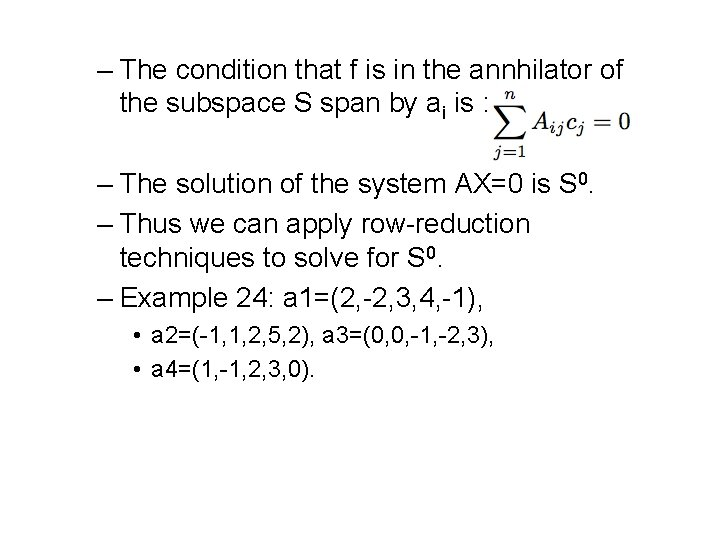– The condition that f is in the annhilator of the subspace S span by ai is : – The solution of the system AX=0 is S 0. – Thus we can apply row-reduction techniques to solve for S 0. – Example 24: a 1=(2, -2, 3, 4, -1), • a 2=(-1, 1, 2, 5, 2), a 3=(0, 0, -1, -2, 3), • a 4=(1, -1, 2, 3, 0).